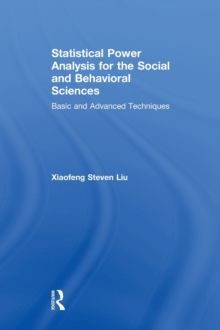Supporting your high street Find out how »
• My Account# Statistical Power Analysis for the Social and Behavioral Sciences : Basic and Advanced Techniques Hardback

#### Description

This is the first book to demonstrate the application of power analysis to the newer more advanced statistical techniques that are increasingly used in the social and behavioral sciences.

Both basic and advanced designs are covered. Readers are shown how to apply power analysis to techniques such as hierarchical linear modeling, meta-analysis, and structural equation modeling.

Each chapter opens with a review of the statistical procedure and then proceeds to derive the power functions.

This is followed by examples that demonstrate how to produce power tables and charts.

The book clearly shows how to calculate power by providing open code for every design and procedure in R, SAS, and SPSS.

Readers can verify the power computation using the computer programs on the book's website.

There is a growing requirement to include power analysis to justify sample sizes in grant proposals.

Most chapters are self-standing and can be read in any order without much disruption.This book will help readers do just that.

Sample computer code in R, SPSS, and SAS at www.routledge.com/9781848729810 are written to tabulate power values and produce power curves that can be included in a grant proposal. Organized according to various techniques, chapters 1 - 3 introduce the basics of statistical power and sample size issues including the historical origin, hypothesis testing, and the use of statistical power in t tests and confidence intervals.

Chapters 4 - 6 cover common statistical procedures -- analysis of variance, linear regression (both simple regression and multiple regression), correlation, analysis of covariance, and multivariate analysis.

Chapters 7 - 11 review the new statistical procedures -- multi-level models, meta-analysis, structural equation models, and longitudinal studies.

The appendixes contain a tutorial about R and show the statistical theory of power analysis.

Intended as a supplement for graduate courses on quantitative methods, multivariate statistics, hierarchical linear modeling (HLM) and/or multilevel modeling and SEM taught in psychology, education, human development, nursing, and social and life sciences, this is the first text on statistical power for advanced procedures.

Researchers and practitioners in these fields also appreciate the book's unique coverage of the use of statistical power analysis to determine sample size in planning a study.

A prerequisite of basic through multivariate statistics is assumed.

#### Information

• Format: Hardback
• Pages: 386 pages, 11 Tables, black and white
• Publisher: Taylor & Francis Ltd
• Publication Date:
• Category: Social research & statistics
• ISBN: 9781848729803

£140.00

£132.15